# 1st PUC Basic Maths Question Bank Chapter 15 Co-ordinate System in a plane

Students can Download Basic Maths Chapter 15 Co-ordinate System in a plane Questions and Answers, Notes Pdf, 1st PUC Basic Maths Question Bank with Answers helps you to revise the complete Karnataka State Board Syllabus and score more marks in your examinations.

## Karnataka 1st PUC Basic Maths Question Bank Chapter 15 Co-ordinate System in a plane

Question 1.
Find the reflection of (-4, 3) through x-axis, y-axis and through the centre.
Reflection through x – axis → (-4, – 3)
Reflection through y – axis → (4,3)
Reflection through center → (4, -3)

Question 2.
Find ‘a’ if the distance between the points (a, 2) and (3, 4) is V8 units.
Let P = (a, 2) and Q = (3, 4)
By data |PQ| = √8
⇒ √8 = $$\sqrt{(3-a)^{2}+(4-2)^{2}}$$
⇒ 8 = (3 – a)2 + 22 = 8
⇒ 9 + a2 – 6a + 4
⇒ a2 – 6a + 5 = 0
⇒ (a – 5)(a – 1) = 0
⇒ a = 5 or a = 1Question 3.
Show that the points (2, 2) (6, 3) and (4,11) form a right angled triangle.
Let A = (2, 2), B = (6, 3), C = (4, 1)
Consider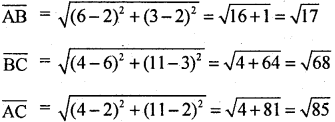By pythagorus theorem AC2 = AB2 + BC2⇒ 85 = 17 + 68 = 85
∴ the points form a right angled triangle.

Question 4.
The points (x, 2) is equidistant from (8, -2) and (2, -2). Find the value of x.
Let A = (2,2) B = (8,-2) C = (2,-2)
By data AB = AC = AB2 = AC2
⇒ (8 – x)2 + (-2 -2)2 = (x – 2)2 + (2 + 2)2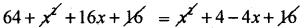-16x + 4x = 4 -64 ⇒ – 12x = -60
∴ x = 5Question 5.
If two vertices of an equilateral triangle are (3,4) and (-2,3). Find the co-ordinates of third vertex.
Let A = (3, 4), B = (-2, 3) and C = (x, y)
bydata AB = BC = CA
⇒ AB2 = BC2 = CA2
consider BC2 = CA2
⇒ (x + 2)2 + (y – 3)2 = (x – 3)2 + (y – 4)24x + 6x – 6y + 8y = 25 – 13
∴ 10x + 2y =12
5x + y = 6 …… (1)
Again AB = BC
⇒ AB2 = BC2
∴ 5(3 + 2)2 + (4 – 3)2 = (x + 2)2 + (y – 3)2 ………. (2)
from(1) y = 6 – 5x
∴ substitute (1) is (2)
(x + 2)2 + (6 – 5x – 3)2 = 26
x2 + 4 + 4x + 9 + 25x2 – 30x = 26
26x2 – 26x – 13 = 0
⇒ 2x2 – 2x – 1 = 0Section Formula

Question 1.
Find the co-ordinates of the point which divide the line joining the points
(i) (1, -3) and (-3, 9) internally in the ratio 1 : 3 and the line joining the points
(ii) (2, -6) (4, 3) externally is the ratio 3 : 2.
(i) Let A = (1, -3) B = (-3 9), Ratio is 1 : 3(ii) Let A = (2, -6) B = (4, 3) ratio 3 : 2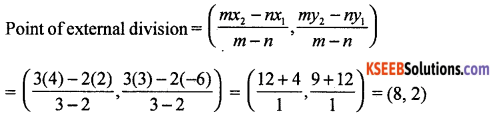Question 2.
Find the ratio in which (2, 7) divides the line joining the point (8, 9) and (-7, 4).
Let
A = (x1 y1) = (8, 9)
B = (x2 y2) = (-7, 4)
p = (x1 y2) = (2, 7)
The ratio l : m in which p divides AB, is given by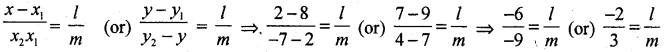⇒ The ratio is l : m = 2 : 3
∴ the points (2,7) divides internallyQuestion 3.
Determine the ratio in which the line 3x + y – 9 = 0 divide the segment joining the points (1, 3) and (2, 7).
Let the of division k : 1 as and point then the coordinates of the points are $$\left(\frac{2 k+1}{k+1}, \frac{7 k+3}{k+1}\right)$$
But the point ‘C’ lies on 3x + y – 9 = 0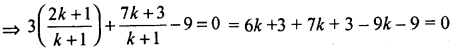⇒ 4x – 3 = 0
⇒ k = $$\frac { 3 }{ 4 }$$
∴ The required ratio is 3 : 4 internally

Question 4.
Find the lengths of the medians of a triangle whose vertices are (3, 5) (5, 3) and (7, 7).